Newton-Raphson Algorithm

Let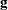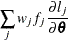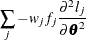be the gradient vector and the Hessian matrix, where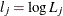is the log likelihood for the jth observation. With a starting value of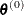, the pseudo-estimate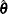ofis obtained iteratively until convergence is obtained: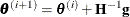whereand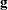are evaluated at the ith iteration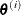. If the log likelihood evaluated at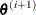is less than that evaluated at, thenis recomputed by step-halving or ridging. The iterative scheme continues until convergence is obtained—that is, untilis sufficiently close to. Then the maximum likelihood estimate ofis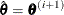.